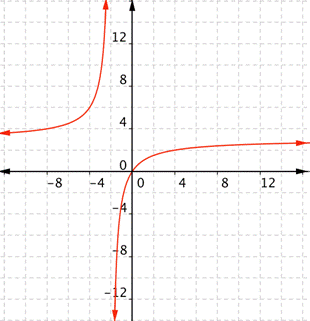## 3.3 – Domain and Range

### Learning Objectives

• (3.3.1) – Define the domain and range of functions from graphs
• (3.3.2) – Define the domain of functions from algebraic expressions

Functions are a correspondence between two sets, called the domain and the range. When defining a function, you usually state what kind of numbers the domain (x) and range (f(x)) values can be. But even if you say they are real numbers, that doesn’t mean that all real numbers can be used for x. It also doesn’t mean that all real numbers can be function values, f(x). There may be restrictions on the domain and range. The restrictions partly depend on the type of function.

In this topic, all functions will be restricted to real number values. That is, only real numbers can be used in the domain, and only real numbers can be in the range.

There are two main reasons why domains are restricted.

• You can’t divide by 0.
• You can’t take the square (or other even) root of a negative number, as the result will not be a real number.

# (3.3.1) – Find Domain and Range From a Graph

Finding domain and range of different functions is often a matter of asking yourself, what values can this function not have? Pictures make it easier to visualize what domain and range are, so we will show how to define the domain and range of functions given their graphs.

What are the domain and range of the real-valued function $f(x)=x+3$?
This is a linear function. Remember that linear functions are lines that continue forever in each direction.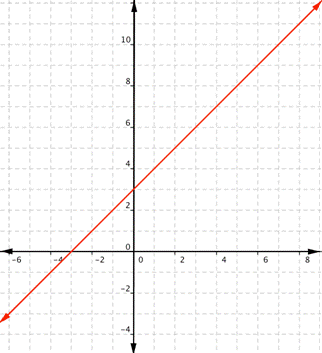Any real number can be substituted for x and get a meaningful output. For any real number, you can always find an x value that gives you that number for the output. Unless a linear function is a constant, such as $f(x)=2$, there is no restriction on the range.
The domain and range are all real numbers.

In the following video we show how to define the domain and range of functions from their graphs.

### Example: Finding Domain and Range from a Graph

Find the domain and range of the function $f$.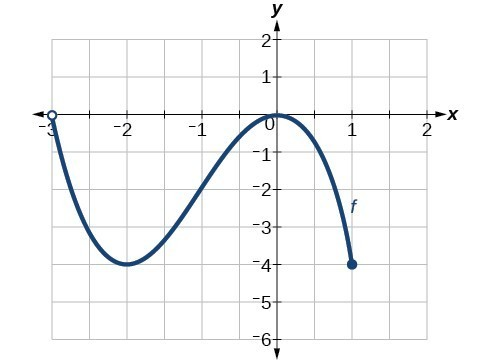### Example: Finding Domain and Range from a Graph of Oil Production

Find the domain and range of the function $f$.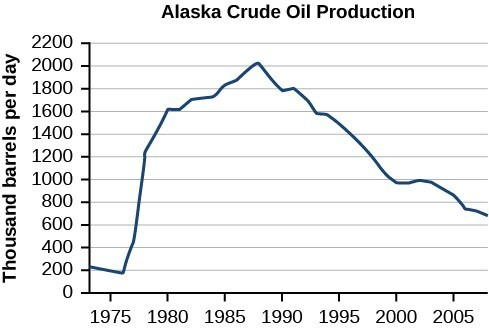(credit: modification of work by the U.S. Energy Information Administration)

### Try It

Given the graph, identify the domain and range using interval notation.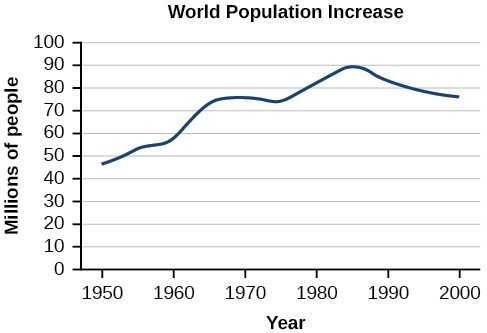# (3.3.2) – Define the domain of functions from algebraic expressions

For the examples that follow, try to figure out the domain and range graphically or algebraically.

### Example

What are the domain and range of the real-valued function $f(x)=−3x^{2}+6x+1$?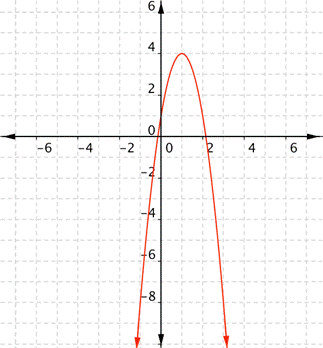### Example

What is the domain and range of the real-valued function $f(x)=-2+\sqrt{x+5}$?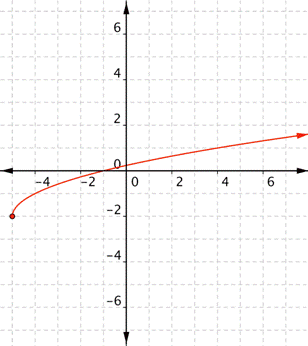Division by 0 could happen whenever the function has a variable in the denominator of a rational expression. That is, it’s something to look for in rational functions. Look at these examples, and note that “division by 0” doesn’t necessarily mean that x is 0! The following is an example of rational function, w will cover these in detail later.

### Example

What is the domain of the real-valued function $\displaystyle f(x)=\frac{3x}{x+2}$?